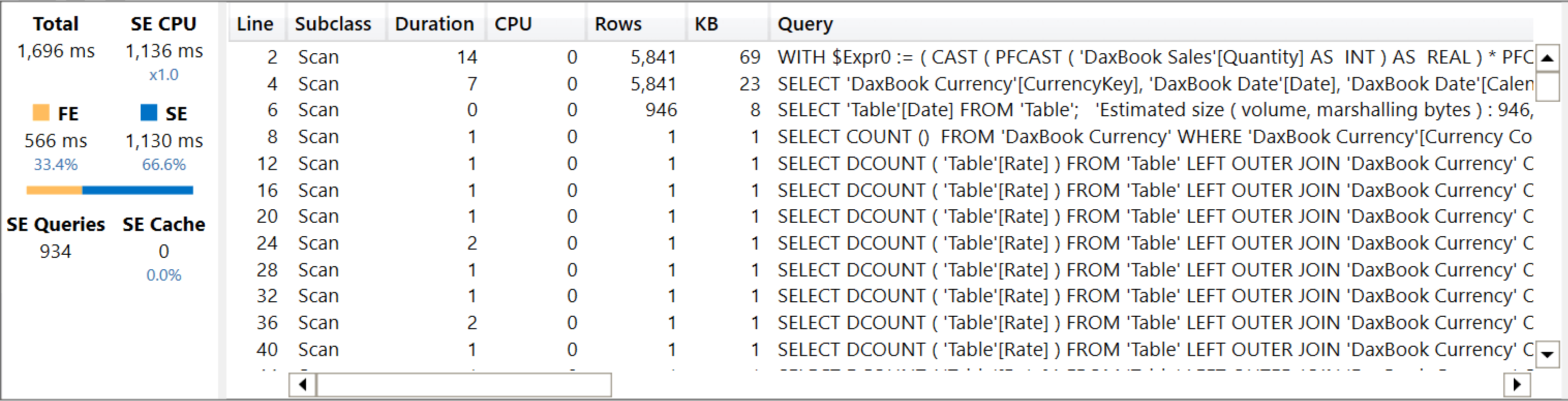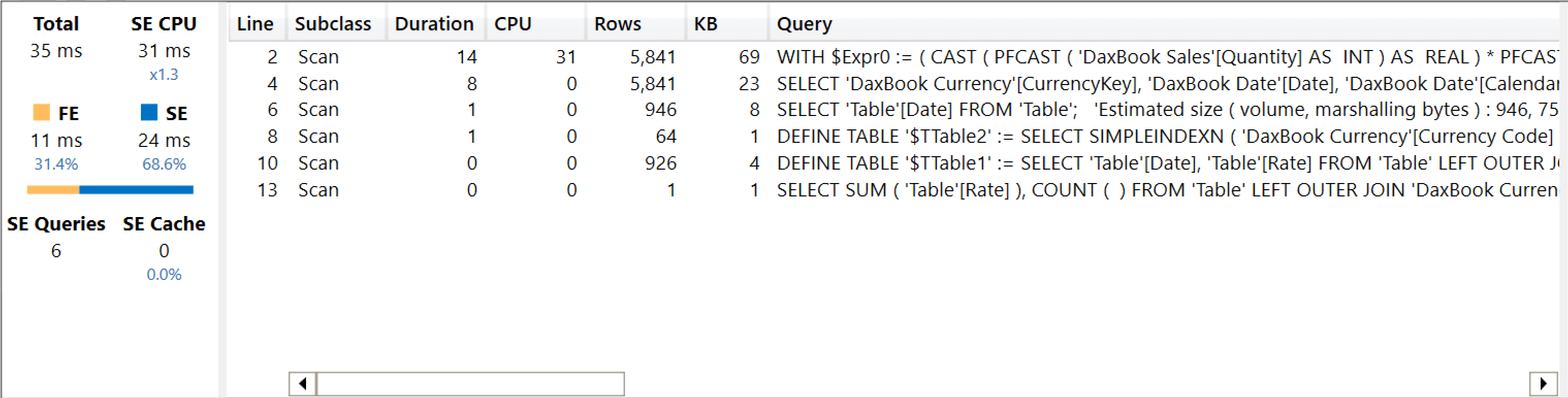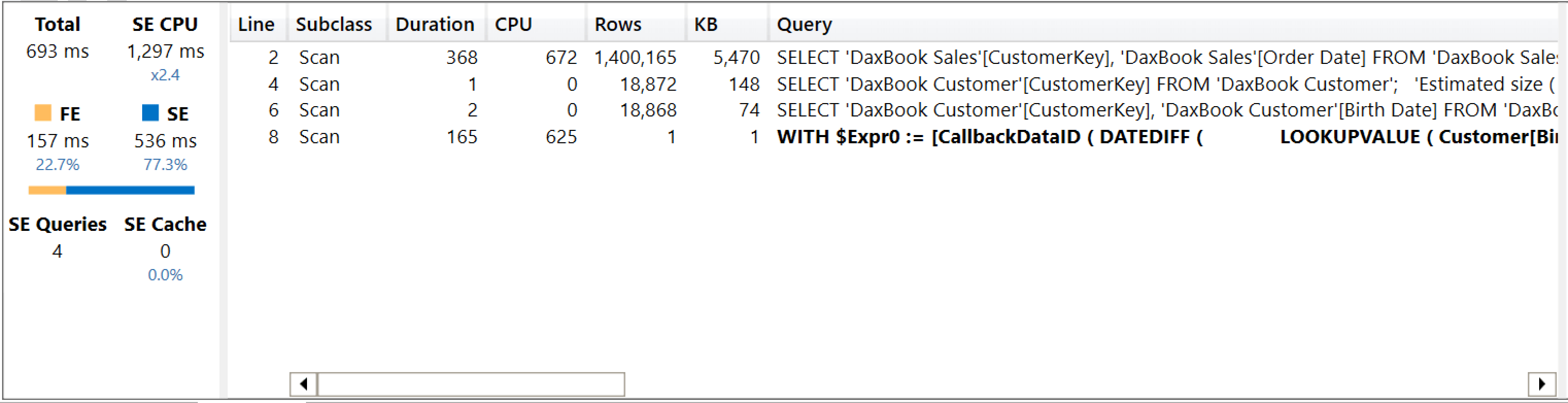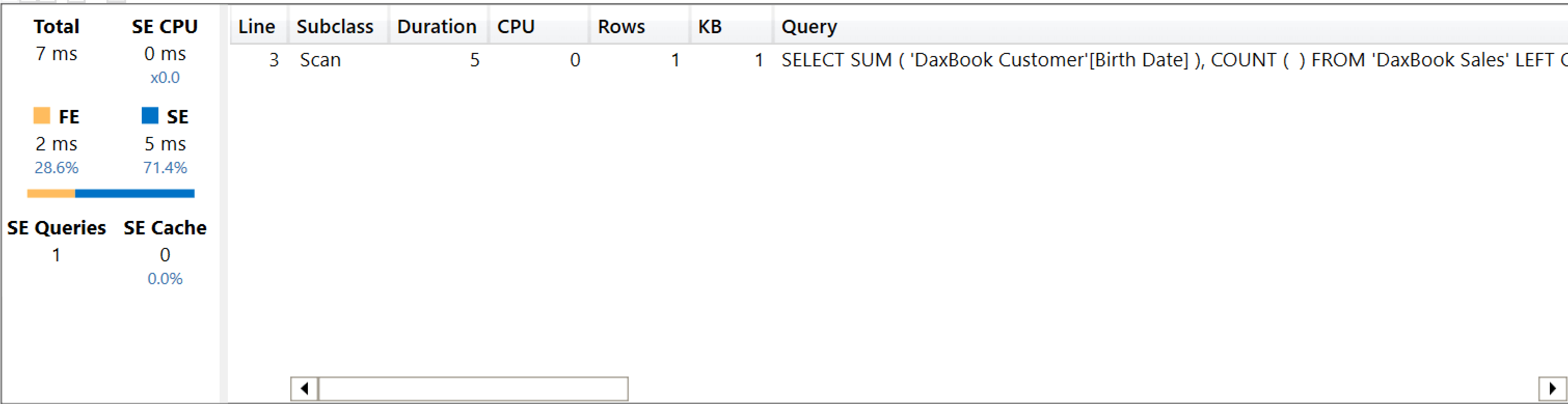If you are searching for an introduction of LOOKUPVALUE, please read the Introducing LOOKUPVALUE article first. Here, we assume that the reader is familiar with the LOOKUPVALUE semantics.

## Internal behavior of LOOKUPVALUE

LOOKUPVALUE requires a column to retrieve a set of column/value pairs to provide the search conditions, and an optional default value in case there are either no matching rows, or too many matching rows. The following formula retrieves the exchange rate from the Daily Exchange Rate table, where Currency[Currency Code] matches EUR and ‘Daily Exchange Rate'[Date] matches Sales[Order Date]. In case there are no matches, it returns zero:

```ExchangeRateToEUR =
VAR CurrentDate = Sales[Order Date]
VAR Result =
LOOKUPVALUE (
'Daily Exchange Rates'[Rate],
'Currency'[Currency Code], "EUR",
'Daily Exchange Rates'[Date], CurrentDate,
0
)
RETURN
Result
```

LOOKUPVALUE is internally translated into a DAX expression that uses more basic functions. The previous code is equivalent to the following:

```ExchangeRateToEUR =
VAR CurrentDate = Sales[Order Date]
VAR Result =
CALCULATE (
SELECTEDVALUE ( 'Daily Exchange Rates'[Rate], 0 ),
FILTER (
ALLNOBLANKROW ( 'Currency'[Currency Code] ),
'Currency'[Currency Code] = "EUR"
),
FILTER (
ALLNOBLANKROW ( 'Daily Exchange Rates'[Date] ),
'Daily Exchange Rates'[Date] = CurrentDate
),
REMOVEFILTERS ( 'Daily Exchange Rates' )
)
RETURN
Result
```

REMOVEFILTERS is important for two reasons. First, LOOKUPVALUE ignores existing filters on the table it is searching. Second, when using LOOKUPVALUE to search in the same table (for example, a calculated column in Sales that searches for another row, still in Sales) you are not affected by circular dependencies that might occur because of CALCULATE.

The presence of SELECTEDVALUE is even more relevant. Indeed, SELECTEDVALUE returns the default value either when there are multiple values in ‘Daily Exchange Rates'[Rate] or when there are none. LOOKUPVALUE without the default value returns an error in case there are multiple values for ‘Daily Exchange Rates'[Rate]. If you provide the default value, then LOOKUPVALUE returns the default value instead of an error.

This behavior produces complex query plans, because the engine checks that there is exactly one value before deciding whether to return the default value or the currently selected value. If your data guarantees that there will be at most one value for the column to return, then a better implementation of the default value is achieved by using COALESCE.

As an example, look at the following query performing currency conversion. In case the exchange rate is missing for a given combination of Date and Currency Code, the formula uses the average over all time as an approximation:

```DEFINE
MEASURE Sales[Amount EUR] =
SUMX (
SUMMARIZE ( Sales, 'Date'[Date] ),
[Sales Amount]
* LOOKUPVALUE (
'Daily Exchange Rates'[Rate],
'Daily Exchange Rates'[Date], 'Date'[Date],
Currency[Currency Code], "EUR",
CALCULATE (
AVERAGE ( 'Daily Exchange Rates'[Rate] ),
Currency[Currency Code] = "EUR",
REMOVEFILTERS ()
)
)
)
EVALUATE
SUMMARIZECOLUMNS (
'Date'[Calendar Year],
"Amount_EUR", 'Sales'[Amount EUR]
)
```

This query runs in 1.7 second on our test machine using a larger model with 12 million rows in the Sales table. This is because of the large number of storage engine (SE) queries executed with the sole purpose of guaranteeing that there is one value for the exchange rate. As you can see, there are 934 SE queries for this simple measure.The same code runs much faster (35 milliseconds) by using COALESCE instead of the default value. It also produces a much better query plan:

```DEFINE
MEASURE Sales[Amount EUR] =
SUMX (
SUMMARIZE ( Sales, 'Date'[Date] ),
[Sales Amount]
* COALESCE (
LOOKUPVALUE (
'Daily Exchange Rates'[Rate],
'Daily Exchange Rates'[Date], 'Date'[Date],
Currency[Currency Code], "EUR"
),
CALCULATE (
AVERAGE ( 'Daily Exchange Rates'[Rate] ),
Currency[Currency Code] = "EUR",
REMOVEFILTERS ()
)
)
)
EVALUATE
SUMMARIZECOLUMNS (
'Date'[Calendar Year],
"Amount_EUR", 'Sales'[Amount EUR]
)
```

Here are the results captured in DAX Studio, showing only 6 SE queries.The latter version of the code produces an error in case there are multiple exchange rates for a given date and currency. If you can guarantee that there are no duplicates whatsoever, then the query plan of the COALESCE version is much more optimized.

Consequently, we advise against using the default value in LOOKUPVALUE, and we recommend using COALESCE instead when possible. As usual, do not take this as a best practice. You always need to measure performance in your model and with your queries. Remember: there are very few golden rules in DAX optimization, and this is not one of them. It is just a suggestion to double check performance before taking for granted that the default result of LOOKUPVALUE is somewhat more optimized.

Beware that the downloadable file we are sharing for this article includes a smaller subset of the full dataset. Therefore, if you test these numbers on your computer, you are very likely to see different results. Still comparable, but rather different.

## Using relationships vs. LOOKUPVALUE

LOOKUPVALUE is a function that should not be widely used in a model. Most of the times, the presence of LOOKUPVALUE in the code is a symptom of a missing relationship, or of a model that could be redesigned more efficiently.

LOOKUPVALUE requires moving filters through CALCULATE, therefore it does not take advantage of the SE feature in the most efficient way. For example, look at the following code where we compute the average age of customers over time. The first version uses LOOKUPVALUE to retrieve customer birth dates:

```EVALUATE
{
AVERAGEX (
Sales,
DATEDIFF (
LOOKUPVALUE ( Customer[Birth Date], Customer[CustomerKey], Sales[CustomerKey] ),
Sales[Order Date],
YEAR
)
)
}
```

As you see from the server timings captured with DAX Studio, the level of materialization is large, and the formula engine (FE) is responsible for most of the execution time.The corresponding query that leverages the existing relationship between Customer and Sales produces a better query plan, where most of the computational effort is pushed down to the SE:

```EVALUATE
{
AVERAGEX (
Sales,
RELATED ( Customer[Birth Date] )
)
}
```

Here are the measurements in DAX Studio, showing only 7ms of execution time.Not only is the query much faster: the important parts are a better usage of the SE, vastly reduced materialization, and the missing CallbackDataIDs. All these factors are a clear indication that a relationship is always preferable over overusing LOOKUPVALUE.

However, there are scenarios where LOOKUPVALUE is useful. For example, in currency conversion patterns, LOOKUPVALUE can be useful to retrieve – at query time – the exchange rate on the order date. That said, some effort in trying to replace LOOKUPVALUE with proper usage of relationships is always a good way to improve performance.

## Using CALCULATE vs. LOOKUPVALUE

Sometimes, it could be useful to rewrite LOOKUPVALUE using more basic functions to force a specific query plan. Beware that these kinds of optimizations are very specific to a model, a data distribution, or a particular query. Therefore, we provide these examples to show that CALCULATE offers more flexibility than LOOKUPVALUE; we are not saying that CALCULATE in general is faster or better than LOOKUPVALUE. Extensive performance testing is always required.

LOOKUPVALUE performs the tests on the columns indicated as search conditions separately. In some scenarios, it is more advisable to apply a filter on multiple columns using a single multi-column filter, or to leverage TREATAS instead of a filter column. LOOKUPVALUE does not offer this opportunity, whereas expanding LOOKUPVALUE to its full implementation with simpler functions lets you modify the filters with more flexibility.

To demonstrate this, we can analyze the query plan of the following query:

```DEFINE
MEASURE Sales[Amount EUR] =
SUMX (
SUMMARIZE ( Sales, 'Date'[Date] ),
[Sales Amount]
* LOOKUPVALUE (
'Daily Exchange Rates'[Rate],
'Daily Exchange Rates'[Date], 'Date'[Date],
Currency[Currency Code], "EUR"
)
)
EVALUATE
SUMMARIZECOLUMNS (
'Date'[Calendar Year],
"Amount_EUR", 'Sales'[Amount EUR]
)
```

LOOKUPVALUE requires two filters: one on the date and one on the currency code. This results in a SE query that looks like this:

```SELECT
'Table'[Date], 'Table'[Rate]
FROM 'Table'
LEFT OUTER JOIN 'Currency' ON 'Table'[CurrencyKey]='Currency'[CurrencyKey]
WHERE
'Table'[Date] IN ( 39955.000000, 39996.000000, 39791.000000, ..[926 total values, not all displayed] )
VAND
'Currency'[Currency Code] ININDEX '\$TTable2'[\$SemijoinProjection];
```

This SE query is identical to the one obtained by a different implementation using CALCULATE and TREATAS; the latter generates a slightly different – and sometimes more efficient – query plan that still relies on the same underlying SE query to retrieve the currency exchange rate:

```DEFINE
MEASURE Sales[Amount EUR] =
SUMX (
SUMMARIZE ( Sales, 'Date'[Date] ),
[Sales Amount]
* CALCULATE (
SELECTEDVALUE ( 'Daily Exchange Rates'[Rate] ),
TREATAS ( { 'Date'[Date] }, 'Daily Exchange Rates'[Date] ),
TREATAS ( { "EUR" }, Currency[Currency Code] )
)
)
EVALUATE
SUMMARIZECOLUMNS (
'Date'[Calendar Year],
"Amount_EUR", 'Sales'[Amount EUR]
)
```

In both cases, the two filter conditions over ‘Daily Exchange Rates'[Date] and Currency[Currency Code] are applied separately and merged with an AND condition in the SE query. TREATAS is well optimized, and in some specific scenarios TREATAS can prevent the appearance of CallbackDataID – consequently reducing the pressure on the FE.

Additional flexibility is possible by using CALCULATE. The DAX query where LOOKUPVALUE is replaced with the full CALCULATE gives you the option of applying the filter using TREATAS with a two-column table containing the pair of date and currency code:

```DEFINE
MEASURE Sales[Amount EUR] =
SUMX (
SUMMARIZE ( Sales, 'Date'[Date] ),
[Sales Amount]
* CALCULATE (
SELECTEDVALUE ( 'Daily Exchange Rates'[Rate] ),
TREATAS (
{ ( 'Date'[Date], "EUR" ) },
'Daily Exchange Rates'[Date],
Currency[Currency Code]
)
)
)
EVALUATE
SUMMARIZECOLUMNS (
'Date'[Calendar Year],
"Amount_EUR", 'Sales'[Amount EUR]
)
```

With this version, the SE query returns the same result as the previous query, but it now filters the columns together, as you can see in the following xmSQL query:

```SELECT
'Currency'[CurrencyKey], 'Date'[Date],
SUM (  ( PFDATAID ( 'Table'[Rate] ) <> 2 )  ),
MIN ( 'Table'[Rate] ),
MAX ( 'Table'[Rate] ),
COUNT (  )
FROM 'Table'
LEFT OUTER JOIN 'Currency' ON 'Table'[CurrencyKey]='Currency'[CurrencyKey]
LEFT OUTER JOIN 'Date' ON 'Table'[Date]='Date'[Date]
WHERE
( 'DaxBook Date'[Date], 'Table'[Date], 'Currency'[Currency Code] ) IN
{
( 39816.000000, 39816.000000, 'EUR' ),
( 39841.000000, 39841.000000, 'EUR' ) ,
( 39669.000000, 39669.000000, 'EUR' ) ,
..[926 total tuples, not all displayed]
};
```

Usually, multi-column filters result in poor performance compared to multiple single-column filters. However, if the number of combinations filtered is very small, a multi-column filter can provide advantages compared to two single-column filters with low selectivity.

This level of optimization is seldom required. Indeed, on our database there are no differences between LOOKUPVALUE and CALCULATE in terms of speed. Nonetheless, with a different data distribution or with a different set of columns to search into, the difference might be relevant. Be mindful: by relevant, we do not mean better. It might also be worse, depending on the peculiarities of the model. Remember to always test these techniques before making a decision on which formula to adopt. Extreme optimization requires extensive testing and a good knowledge of the internals of the DAX engine.

LOOKUPVALUERetrieves a value from a table.

`LOOKUPVALUE ( <Result_ColumnName>, <Search_ColumnName>, <Search_Value> [, <Search_ColumnName>, <Search_Value> [, … ] ] [, <Alternate_Result>] )`

REMOVEFILTERSCALCULATE modifier

Clear filters from the specified tables or columns.

`REMOVEFILTERS ( [<TableNameOrColumnName>] [, <ColumnName> [, <ColumnName> [, … ] ] ] )`

CALCULATEContext transition

Evaluates an expression in a context modified by filters.

`CALCULATE ( <Expression> [, <Filter> [, <Filter> [, … ] ] ] )`

SELECTEDVALUEReturns the value when there’s only one value in the specified column, otherwise returns the alternate result.

`SELECTEDVALUE ( <ColumnName> [, <AlternateResult>] )`

COALESCEReturns the first argument that does not evaluate to a blank value. If all arguments evaluate to blank values, BLANK is returned.

`COALESCE ( <Value1>, <Value2> [, <Value2> [, … ] ] )`

TREATASTreats the columns of the input table as columns from other tables.For each column, filters out any values that are not present in its respective output column.

`TREATAS ( <Expression>, <ColumnName> [, <ColumnName> [, … ] ] )`

ANDChecks whether all arguments are TRUE, and returns TRUE if all arguments are TRUE.

`AND ( <Logical1>, <Logical2> )`